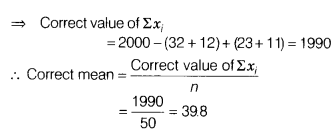# The mean of 50 items was found to be 40. If at the time of calculation two items

1. The mean of 50 items was found to be 40. If at the time of calculation two items were wrongly taken as 32 and 12 instead of 23 and 11, then find the correct mean.